# Trigonometric Pattern Design

Triangles are my favorite shape, three points where two lines meet (Tessellate, Alt-J)

Inspired by recurrence plots and by the Gauss error function, I have done the following plots. The first one represents the recurrence plot of$f\left ( x \right )= sec\left ( x \right )$ where distance between points is measured by Gauss error function:This one is the same for$f\left ( x \right )= tag\left ( x \right )$And this one represents$latex f\left ( x \right )= sin\left ( x \right )$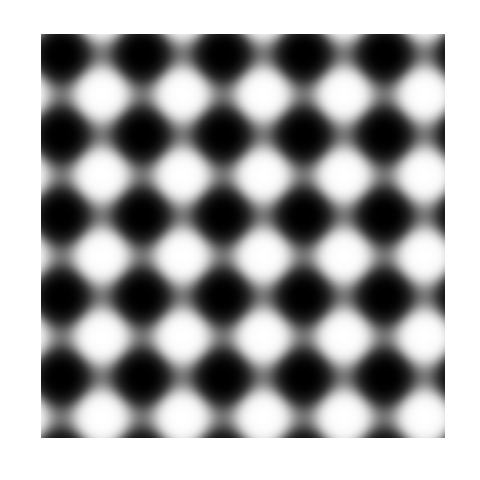I like them: they are elegant, attractive and easy to make. Try your own functions. One final though: the more I use magrittr package, the more I like it. This is the code for the first plot.

library("magrittr")
library("ggplot2")
library("pracma")
RecurrencePlot = function(from, to, col1, col2) {
opt = theme(legend.position  = "none",
panel.background = element_blank(),
axis.ticks       = element_blank(),
panel.grid       = element_blank(),
axis.title       = element_blank(),
axis.text        = element_blank())
seq(from, to, by = .1) %>% expand.grid(x=., y=.) %>%
ggplot( ., aes(x=x, y=y, fill=erf(sec(x)-sec(y)))) + geom_tile() +
RecurrencePlot(from = -5*pi, to = 5*pi, col1 = "black", col2= "white")


# Shiny Wool Skeins

Chaos is not a pit: chaos is a ladder (Littlefinger in Game of Thrones)

Some time ago I wrote this post to show how my colleague Vu Anh translated into Shiny one of my experiments, opening my eyes to an amazing new world. I am very proud to present you the first Shiny experiment entirely written by me.

In this case I took inspiration from another previous experiment to draw some kind of wool skeins. The shiny app creates a plot consisting of chords inside a circle. There are to kind of chords:

• Those which form a track because they are a set of glued chords; number of tracks and number of chords per track can be selected using Number of track chords and Number of scrawls per track sliders of the app respectively.
• Those forming the background, randomly allocated inside the circle. Number of background chords can be chosen as well in the app

There is also the possibility to change colors of chords. This are the main steps I followed to build this Shiny app:

1. Write a simple R program
2. Decide which variables to parametrize
3. Open a new Shiny project in RStudio
4. Analize the sample UI.R and server.R files generated by default
5. Adapt sample code to my particular code (some iterations are needed here)
6. Deploy my app in the Shiny Apps free server

Number 1 is the most difficult step, but it does not depends on Shiny: rest of them are easier, specially if you have help as I had from my colleague Jorge. I encourage you to try. This is an snapshot of the app:You can play with the app here.

Some things I thought while developing this experiment:

• Shiny gives you a lot with a minimal effort
• Shiny can be a very interesting tool to teach maths and programming to kids
• I have to translate to Shiny some other experiment
• I will try to use it for my job

Try Shiny: is very entertaining. A typical Shiny project consists on two files, one to define the user interface (UI.R) and the other to define the back end side (server.R).

This is the code of UI.R:

# This is the user-interface definition of a Shiny web application.
# You can find out more about building applications with Shiny here:
#
# http://shiny.rstudio.com
#

library(shiny)

shinyUI(fluidPage(

# Application title
titlePanel("Shiny Wool Skeins"),
HTML("

This experiment is based on <a href=\"https://aschinchon.wordpress.com/2015/05/13/bertrand-or-the-importance-of-defining-problems-properly/\">this previous one</a> I did some time ago. It is my second approach to the wonderful world of Shiny.

"),
# Sidebar with a slider input for number of bins
sidebarLayout(
sidebarPanel(
inputPanel(
sliderInput("lin", label = "Number of track chords:",
min = 1, max = 20, value = 5, step = 1),
sliderInput("rep", label = "Number of scrawls per track:",
min = 1, max = 50, value = 10, step = 1),
sliderInput("nbc", label = "Number of background chords:",
min = 0, max = 2000, value = 500, step = 2),
selectInput("col1", label = "Track colour:",
choices = colors(), selected = "darkmagenta"),
selectInput("col2", label = "Background chords colour:",
choices = colors(), selected = "gold")
)

),

# Show a plot of the generated distribution
mainPanel(
plotOutput("chordplot")
)
)
))


And this is the code of server.R:

# This is the server logic for a Shiny web application.
# You can find out more about building applications with Shiny here:
#
# http://shiny.rstudio.com
#
library(ggplot2)
library(magrittr)
library(grDevices)
library(shiny)

shinyServer(function(input, output) {

df<-reactive({ ini=runif(n=input$lin, min=0,max=2*pi) data.frame(ini=runif(n=input$lin, min=0,max=2*pi),
end=runif(n=input$lin, min=pi/2,max=3*pi/2)) -> Sub1 Sub1=Sub1[rep(seq_len(nrow(Sub1)), input$rep),]
Sub1 %>% apply(c(1, 2), jitter) %>% as.data.frame() -> Sub1
Sub1=with(Sub1, data.frame(col=input$col1, x1=cos(ini), y1=sin(ini), x2=cos(end), y2=sin(end))) Sub2=runif(input$nbc, min = 0, max = 2*pi)
Sub2=data.frame(x=cos(Sub2), y=sin(Sub2))
Sub2=cbind(input$col2, Sub2[(1:(input$nbc/2)),], Sub2[(((input$nbc/2)+1):input$nbc),])
colnames(Sub2)=c("col", "x1", "y1", "x2", "y2")
rbind(Sub1, Sub2)
})

opts=theme(legend.position="none",
panel.background = element_rect(fill="white"),
panel.grid = element_blank(),
axis.ticks=element_blank(),
axis.title=element_blank(),
axis.text =element_blank())

output$chordplot<-renderPlot({ p=ggplot(df())+geom_segment(aes(x=x1, y=y1, xend=x2, yend=y2), colour=df()$col, alpha=runif(nrow(df()), min=.1, max=.3), lwd=1)+opts;print(p)
}, height = 600, width = 600 )
})


# Odd Connections Inside The NASDAQ-100

Distinguishing the signal from the noise requires both scientific knowledge and self-knowledge (Nate Silver, author of The Signal and the Noise)

Analyzing the evolution of NASDAQ-100 stock prices can discover some interesting couples of companies which share a strong common trend despite of belonging to very different sectors. The NASDAQ-100 is made up of 107 equity securities issued by 100 of the largest non-financial companies listed on the NASDAQ. On the other side, Yahoo! Finance is one of the most popular services to consult financial news, data and commentary including stock quotes, press releases, financial reports, and original programming. Using R is possible to download the evolution of NASDAQ-100 symbols from Yahoo! Finance. There is a R package called quantmod which makes this issue quite simple with the function getSymbols. Daily series are long enough to do a wide range of analysis, since most of them start in 2007.

One robust way to determine if two times series, xt and yt, are related is to analyze if there exists an equation like yt=βxt+ut such us residuals (ut) are stationary (its mean and variance does not change when shifted in time). If this happens, it is said that both series are cointegrated. The way to measure it in R is running the Augmented Dickey-Fuller test, available in tseries package. Cointegration analysis help traders to design products such spreads and hedges.

There are 5.671 different couples between the 107 stocks of NASDAQ-100. After computing the Augmented Dickey-Fuller test to each of them, the resulting data frame can be converted into a distance matrix. A nice way to visualize distances between stocks is to do a hierarchical clustering. This is the resulting dendogram of the clustering:Close stocks such as Ca Inc. (CA) and Bed Bath & Beyond Inc. (BBBY) are joined with short links. A quick way to extract close couples is to cut this dendogram in a big number of clusters and keep those with two elements. Following is the list of the most related stock couples cutting dendogram in 85 clusters: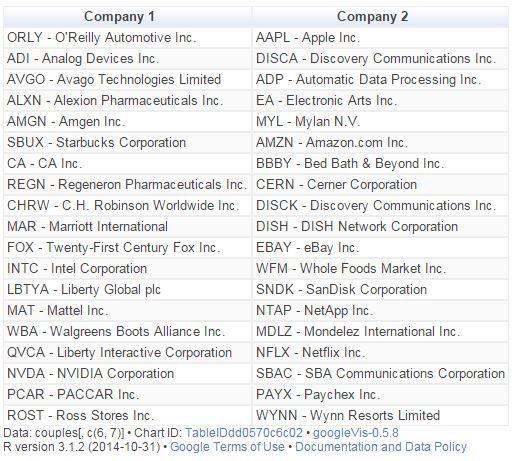Most of them are strange neighbors. Next plot shows the evolution closing price evolution of four of these couples: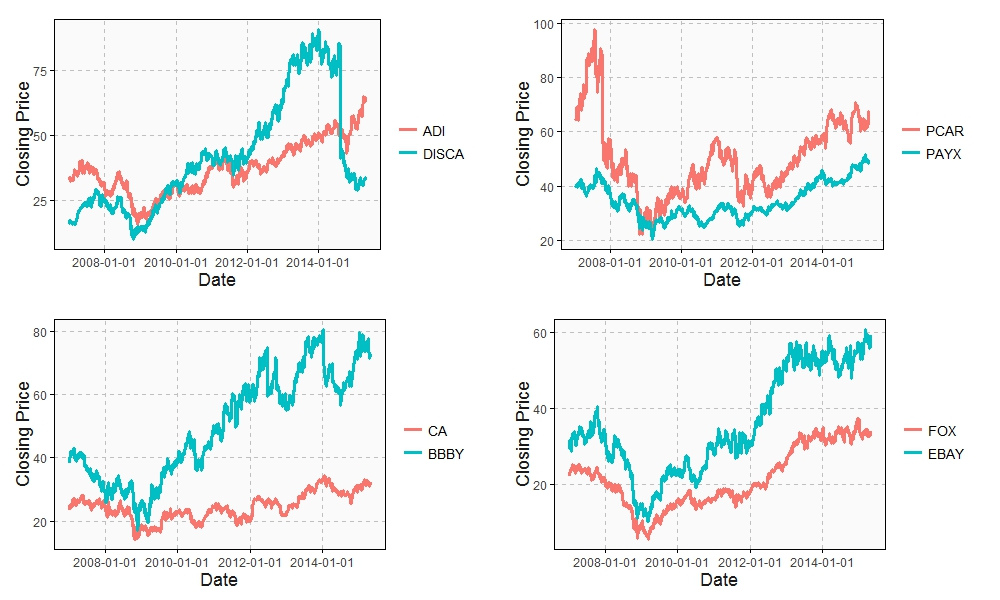Analog Devices Inc. (ADI) makes semiconductors and Discovery Communications Inc. (DISCA) is a mass media company. PACCAR Inc. (PCAR) manufactures trucks and Paychex Inc. (PAYX) provides HR outsourcing. CA Inc. (CA) creates software and Bed Bath & Beyond Inc. (BBBY) sells goods for home. Twenty-First Century Fox Inc. (FOX) is a mass media company as well and EBAY Inc. (EBAY) does online auctions‎. All of them are odd connections.

This is the code of the experiment:

library("quantmod")
library("TSdist")
library("ggplot2")
library("Hmisc")
library("zoo")
library("scales")
library("reshape2")
library("tseries")
library("RColorBrewer")
library("ape")
library("sqldf")
library("gridExtra")
setwd("YOUR-WORKING-DIRECTORY-HERE")
temp=tempfile()
for (i in 1:nrow(data)) getSymbols(as.character(data[i,1]))
results=t(apply(combn(sort(as.character(data[,1]), decreasing = TRUE), 2), 2,
function(x) {
ts1=drop(Cl(eval(parse(text=x))))
ts2=drop(Cl(eval(parse(text=x))))
t.zoo=merge(ts1, ts2, all=FALSE)
t=as.data.frame(t.zoo)
m=lm(ts2 ~ ts1 + 0, data=t)
beta=coef(m)
sprd=t$ts1 - beta*t$ts2
ht=adf.test(sprd, alternative="stationary", k=0)$p.value c(symbol1=x, symbol2=x, (1-ht))})) results=as.data.frame(results) colnames(results)=c("Sym1", "Sym2", "TSdist") results$TSdist=as.numeric(as.character(results$TSdist)) save(results, file="results.RData") load("results.RData") m=as.dist(acast(results, Sym1~Sym2, value.var="TSdist")) hc = hclust(m) # vector of colors op = par(bg = "darkorchid4") plot(as.phylo(hc), type = "fan", tip.color = "gold", edge.color ="gold", cex=.8) # cutting dendrogram in 85 clusters clusdf=data.frame(Symbol=names(cutree(hc, 85)), clus=cutree(hc, 85)) clusdf2=merge(clusdf, data[,c(1,2)], by="Symbol") sizes=sqldf("SELECT * FROM (SELECT clus, count(*) as size FROM clusdf GROUP BY 1) as T00 WHERE size>=2") sizes2=merge(subset(sizes, size==2), clusdf2, by="clus") sizes2$id=sequence(rle(sizes2$clus)$lengths)
couples=merge(subset(sizes2, id==1)[,c(1,3,4)], subset(sizes2, id==2)[,c(1,3,4)], by="clus")
couples$"Company 1"=apply(couples[ , c(2,3) ] , 1 , paste , collapse = " -" ) couples$"Company 2"=apply(couples[ , c(4,5) ] , 1 , paste , collapse = " -" )
CouplesTable=gvisTable(couples[,c(6,7)])
plot(CouplesTable)
# Plots
opts2=theme(
panel.background = element_rect(fill="gray98"),
panel.border = element_rect(colour="black", fill=NA),
axis.line = element_line(size = 0.5, colour = "black"),
axis.ticks = element_line(colour="black"),
panel.grid.major = element_line(colour="gray75", linetype = 2),
panel.grid.minor = element_blank(),
axis.text = element_text(colour="gray25", size=12),
axis.title = element_text(size=18, colour="gray10"),
legend.key = element_rect(fill = "white"),
legend.text = element_text(size = 14),
legend.background = element_rect(),
plot.title = element_text(size = 35, colour="gray10"))
plotPair = function(Symbol1, Symbol2)
{
getSymbols(Symbol1)
getSymbols(Symbol2)
close1=Cl(eval(parse(text=Symbol1)))
close2=Cl(eval(parse(text=Symbol2)))
cls=merge(close1, close2, all = FALSE)
df=data.frame(date = time(cls), coredata(cls))
names(df)[-1]=c(Symbol1, Symbol2)
df1=melt(df, id.vars = "date", measure.vars = c(Symbol1, Symbol2))
ggplot(df1, aes(x = date, y = value, color = variable))+
geom_line(size = I(1.2))+
scale_color_discrete(name = "")+
scale_x_date(labels = date_format("%Y-%m-%d"))+
labs(x="Date", y="Closing Price")+
opts2
}
p2=plotPair("PCAR", "PAYX")
p3=plotPair("CA", "BBBY")
p4=plotPair("FOX", "EBAY")
grid.arrange(p1, p2, p3, p4, ncol=2)


# NASDAQ 100 Couples

Heaven, I’m in heaven, and my heart beats so that I can hardly speak, and I seem to find the happiness I seek, when we’re out together dancing cheek to cheek (Cheek To Cheek, Irving Berlin)

There are about 6.500 available packages in CRAN repository. If I were a superhuman, able to learn one package a day, I would spend almost 18 years of my life studying R. And how many packages would be uploaded to CRAN during this period? Who knows: R is infinite.

Today, my experiment deals with quantmod package, which allows you to play to be quant for a while. I download the daily quotes of NASDAQ 100 companies and measure distances between each pair of companies. Distance is based on the cross-correlation between two series so high-correlated series (not exceeding a maximum lag) are closer than low-correlated ones. You can read a good description of this distance here. Since NASDAQ 100 contains 107 companies, I calculate distances for 5.671 different couples. Next plot represent distances between each pair of companies. The darker is the color, the closer are the related companies:Yes, I know is not a graph for someone with visual problems. Let me show you an example of what is behind one of these little tiles. Distance between Mattel Inc. and 21st Century Fox is very small (its related tile is dark coloured). Why? Because of this:These two companies have been dancing cheek to cheek for more than seven years. It is also curious how some companies are far from any of their NASDAQ 100 colleagues. Some examples of these unpaired companies are Express Scripts Holding Company (ESRX), Expeditors International of Washington Inc. (EXPD) and Fastenal Company (FAST). I do not why but there must be an explanation, do not you think so?

Something tells me I will do some other experiment using quantmod package:

library("quantmod")
library("TSdist")
library("ggplot2")
library("Hmisc")
library("zoo")
library("scales")
library("reshape2")
temp=tempfile()
for (i in 1:nrow(data)) getSymbols(as.character(data[i,1]))
results=t(apply(combn(sort(as.character(data[,1]), decreasing = TRUE), 2), 2,
function(x)
{
ts1=drop(Cl(eval(parse(text=x))))
ts2=drop(Cl(eval(parse(text=x))))
c(symbol1=x, symbol2=x, tsDistances(ts1, ts2, distance="crosscorrelation"))
}))
results=as.data.frame(results)
colnames(results)=c("Sym1", "Sym2", "TSdist")
results$TSdist=as.numeric(as.character(results$TSdist))
results=rbind(results, data.frame(Sym1=as.character(data[,1]), Sym2=as.character(data[,1]), TSdist=0))
results$TSdist2=as.numeric(cut2(results$TSdist, g=4))
opts=theme(axis.text.x = element_text(angle = 90, vjust=.5, hjust = 0),
panel.background = element_blank(),
axis.text = element_text(colour="gray25", size=8),
legend.position = "none",
panel.grid = element_blank())
ggplot(results,aes(x=Sym2,y=Sym1))+
geom_tile(aes(fill = TSdist2), colour="gray80")+
scale_size_continuous(range=c(1,10))+
scale_x_discrete("", limits=sort(unique(as.character(results$Sym1))))+ scale_y_discrete("", limits=sort(unique(as.character(results$Sym2)), decreasing = TRUE))+
scale_fill_gradient(low = "steelblue", high = "white")+
opts
MAT.close=Cl(MAT)
FOX.close=Cl(FOX)
cls=merge(MAT.close, FOX.close, all = FALSE)
df=data.frame(date = time(cls), coredata(cls))
names(df)[-1]=c("mat", "fox")
df1=melt(df, id.vars = "date", measure.vars = c("mat", "fox"))
opts2=theme(
panel.background = element_rect(fill="gray98"),
panel.border = element_rect(colour="black", fill=NA),
axis.line = element_line(size = 0.5, colour = "black"),
axis.ticks = element_line(colour="black"),
panel.grid.major = element_line(colour="gray75", linetype = 2),
panel.grid.minor = element_blank(),
axis.text = element_text(colour="gray25", size=15),
axis.title = element_text(size=18, colour="gray10"),
legend.key = element_blank(),
legend.position = "none",
legend.background = element_blank(),
plot.title = element_text(size = 40, colour="gray10"))
ggplot(df1, aes(x = date, y = value, color = variable))+
geom_line(size = I(1.2))+
scale_color_discrete(guide = "none")+
scale_x_date(labels = date_format("%Y-%m-%d"))+
labs(title="Nasdaq 100 Couples: Mattel And Fox", x="Date", y="Closing Price")+
annotate("text", x = as.Date("2011-01-01", "%Y-%m-%d"), y = c(10, 30), label = c("21st Century Fox", "Mattel Inc."), size=7, colour="gray25")+
opts2


# The World We Live In #4: Marriage Ages

It is time for women to stop being politely angry (Leymah Gbowee, Nobel Prize Peace Winner)

Sometimes very simple plots give insight into we live in a world of differences. This plot shows the mean age at marriage for men and women across countries: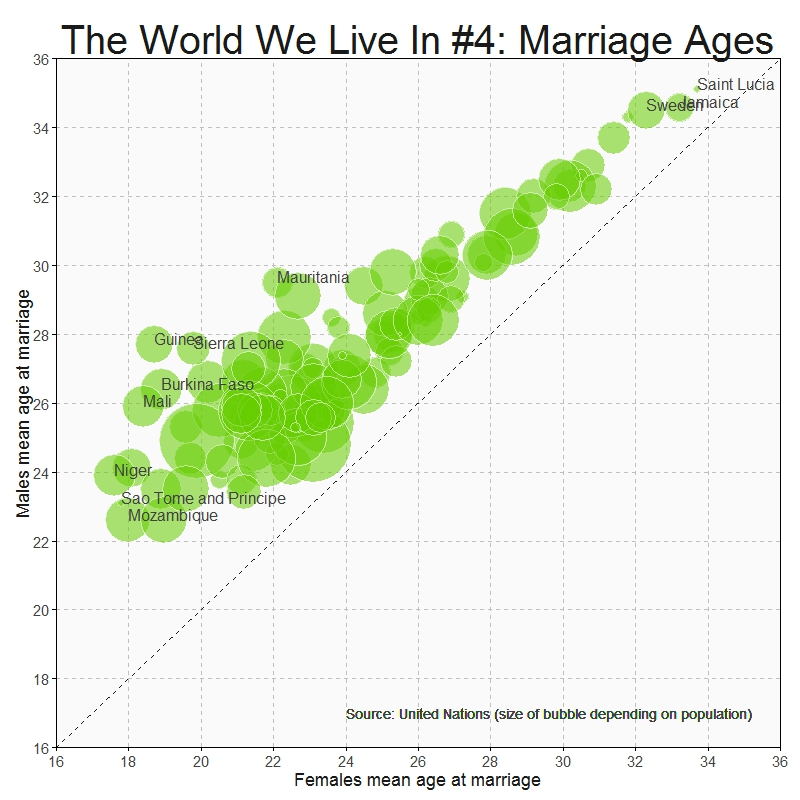Being a woman in some countries of this world must be a hard experience:

#Singulate mean age at marriage: http://data.un.org/Data.aspx?d=GenderStat&f=inID%3a20
#Population: http://data.un.org/Data.aspx?d=SOWC&f=inID%3a105
require("sqldf")
require("ggplot2")
colnames(mar)="Country"
colnames(pop)="Country"
data=sqldf("SELECT
a.Country,
a.Value as Pop,
b.Value as Female,
c.Value as Male
FROM
pop a INNER JOIN mar b
ON (a.Country=b.Country AND b.Subgroup='Female') INNER JOIN mar c
ON (a.Country=c.Country AND c.Subgroup='Male')
WHERE a.Subgroup = 'Total'")
opts=theme(
panel.background = element_rect(fill="gray98"),
panel.border = element_rect(colour="black", fill=NA),
axis.line = element_line(size = 0.5, colour = "black"),
axis.ticks = element_line(colour="black"),
panel.grid.major = element_line(colour="gray75", linetype = 2),
panel.grid.minor = element_blank(),
axis.text = element_text(colour="gray25", size=15),
axis.title = element_text(size=18, colour="gray10"),
legend.key = element_blank(),
legend.position = "none",
legend.background = element_blank(),
plot.title = element_text(size = 40, colour="gray10"))
ggplot(data, aes(x=Female, y=Male, size=log(Pop), label=Country), guide=FALSE)+
geom_point(colour="white", fill="chartreuse3", shape=21, alpha=.55)+
scale_size_continuous(range=c(2,36))+
scale_x_continuous(limits=c(16,36), breaks=seq(16, 36, by = 2), expand = c(0, 0))+
scale_y_continuous(limits=c(16,36), breaks=seq(16, 36, by = 2), expand = c(0, 0))+
geom_abline(intercept = 0, slope = 1, colour = "gray10", linetype=2)+
labs(title="The World We Live In #4: Marriage Ages",
x="Females mean age at marriage",
y="Males mean age at marriage")+
geom_text(data=subset(data, abs(Female-Male)>7), size=5.5, colour="gray25", hjust=0, vjust=0)+
geom_text(data=subset(data, Female>=32|Female<=18), size=5.5, colour="gray25", hjust=0, vjust=0)+
geom_text(aes(24, 17), colour="gray25", hjust=0, label="Source: United Nations (size of bubble depending on population)", size=5)+opts


# Visual Complexity

Oh, can it be, the voices calling me, they get lost and out of time (Little Black Submarines, The Black Keys)

Last October I did this experiment about complex domain coloring. Since I like giving my posts a touch of randomness, I have done this experiment. I plot four random functions on the form p1(x)*p2(x)/p3(x) where pi(x) are polynomials up-to-4th-grade with random coefficients following a chi-square distribution with degrees of freedom between 2 and 5. I measure the function over the complex plane and arrange the four resulting plots into a 2×2 grid. This is an example of the output:Every time you run the code you will obtain a completely different output. I have run it hundreds of times because results are always surprising. Do you want to try? Do not hesitate to send me your creations. What if you change the form of the functions or the distribution of coefficients? You can find my email here.

setwd("YOUR WORKING DIRECTORY HERE")
require(polynom)
require(ggplot2)
library(gridExtra)
ncol=2
for (i in 1:(10*ncol)) {eval(parse(text=paste("p",formatC(i, width=3, flag="0"),"=as.function(polynomial(rchisq(n=sample(2:5,1), df=sample(2:5,1))))",sep="")))}
z=as.vector(outer(seq(-5, 5, by =.02),1i*seq(-5, 5, by =.02),'+'))
opt=theme(legend.position="none",
panel.background = element_blank(),
panel.margin = unit(0,"null"),
panel.grid = element_blank(),
axis.ticks= element_blank(),
axis.title= element_blank(),
axis.text = element_blank(),
strip.text =element_blank(),
axis.ticks.length = unit(0,"null"),
axis.ticks.margin = unit(0,"null"),
plot.margin = rep(unit(0,"null"),4))
for (i in 1:(ncol^2))
{
pols=sample(1:(10*ncol), 3, replace=FALSE)
p1=paste("p", formatC(pols, width=3, flag="0"), "(x)*", sep="")
p2=paste("p", formatC(pols, width=3, flag="0"), "(x)/", sep="")
p3=paste("p", formatC(pols, width=3, flag="0"), "(x)",  sep="")
eval(parse(text=paste("p = function (x) ", p1, p2, p3, sep="")))
df=data.frame(x=Re(z),
y=Im(z),
h=(Arg(p(z))<0)*1+Arg(p(z))/(2*pi),
s=(1+sin(2*pi*log(1+Mod(p(z)))))/2,
v=(1+cos(2*pi*log(1+Mod(p(z)))))/2)
g=ggplot(data=df[is.finite(apply(df,1,sum)),], aes(x=x, y=y)) + geom_tile(fill=hsv(df$h,df$s,df$v))+ opt assign(paste("hsv_g", formatC(i, width=3, flag="0"), sep=""), g) } jpeg(filename = "Surrealism.jpg", width = 800, height = 800, quality = 100) grid.arrange(hsv_g001, hsv_g002, hsv_g003, hsv_g004, ncol=ncol) dev.off()  # Silhouettes Romeo, Juliet, balcony in silhouette, makin o’s with her cigarette, it’s juliet (Flapper Girl, The Lumineers) Two weeks ago I published this post for which designed two different visualizations. At the end, I decided to place words on the map of the United States. The discarded visualization was this other one, where I place the words over the silhouette of each state: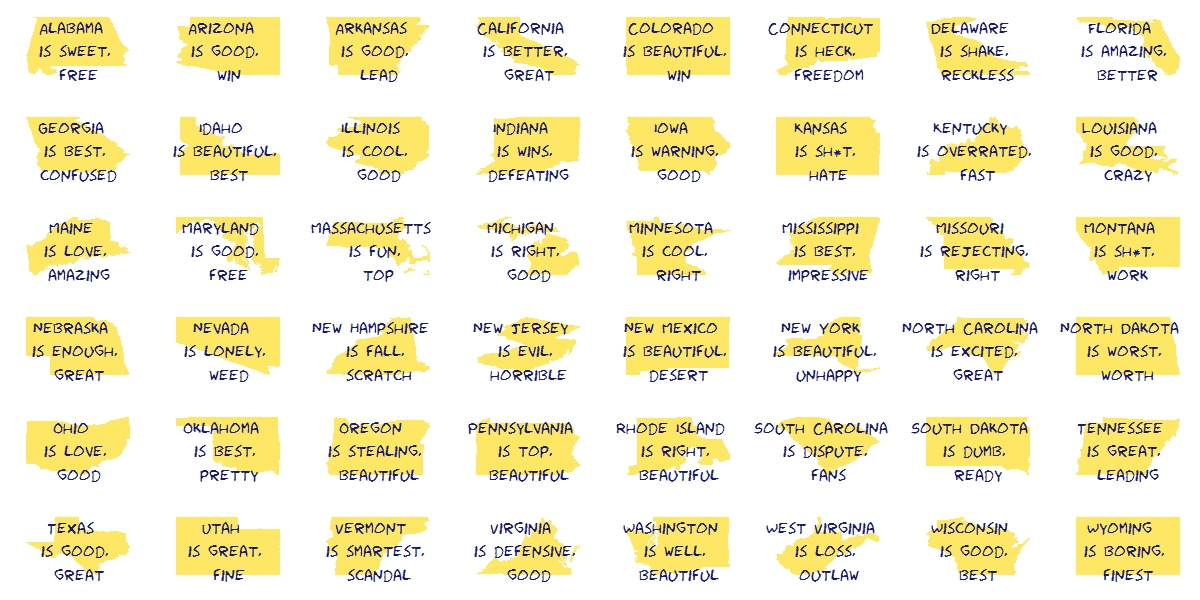I do not want to set aside this chart because I really like it and also because I think it is a nice example of the possibilities one have working with R. Here you have the code. It substitutes the fragment of the code headed by “Visualization” of the original post: library(ggplot2) library(maps) library(gridExtra) library(extrafont) opt=theme(legend.position="none", panel.background = element_blank(), panel.grid = element_blank(), axis.ticks=element_blank(), axis.title=element_blank(), axis.text =element_blank(), plot.title = element_text(size = 28)) vplayout=function(x, y) viewport(layout.pos.row = x, layout.pos.col = y) grid.newpage() jpeg(filename = "States In Two Words.jpeg", width = 1200, height = 600, quality = 100) pushViewport(viewport(layout = grid.layout(6, 8))) for (i in 1:nrow(table)) { wd=subset(words, State==as.character(table$"State name"[i]))
p=ggplot() + geom_polygon( data=subset(map_data("state"), region==tolower(table$"State name"[i])), aes(x=long, y=lat, group = group), colour="white", fill="gold", alpha=0.6, linetype=0 )+opt print(p, vp = vplayout(floor((i-1)/8)+1, i%%8+(i%%8==0)*8)) txt=paste(as.character(table$"State name"[i]),"\n is", wd$word1,"\n and", wd$word2, sep=" ")
grid.text(txt, gp=gpar(font=1, fontsize=16, col="midnightblue", fontfamily="Humor Sans"), vp = viewport(layout.pos.row = floor((i-1)/8)+1, layout.pos.col = i%%8+(i%%8==0)*8))
}
dev.off()


A technique succeeds in mathematical physics, not by a clever trick, or a happy accident, but because it expresses some aspect of physical truth (O. G. Sutton)

Imagine three unbalanced coins:

• Coin 1: Probability of head=0.495 and probability of tail=0.505
• Coin 2: Probability of head=0.745 and probability of tail=0.255
• Coin 3: Probability of head=0.095 and probability of tail=0.905

Now let’s define two games using these coins:

• Game A: You toss coin 1 and if it comes up head you receive 1€ but if not, you lose 1€
• Game B: If your present capital is a multiple of 3, you toss coin 2. If not, you toss coin 3. In both cases, you receive 1€ if coin comes up head and lose 1€ if not.

Played separately, both games are quite unfavorable. Now let’s define Game A+B in which you toss a balanced coin and if it comes up head, you play Game A and play Game B otherwise. In other words, in Game A+B you decide between playing Game A or Game B randomly.

Starting with 0€, it is easy to simulate the three games along 500 plays. This is an example of one of these simulations: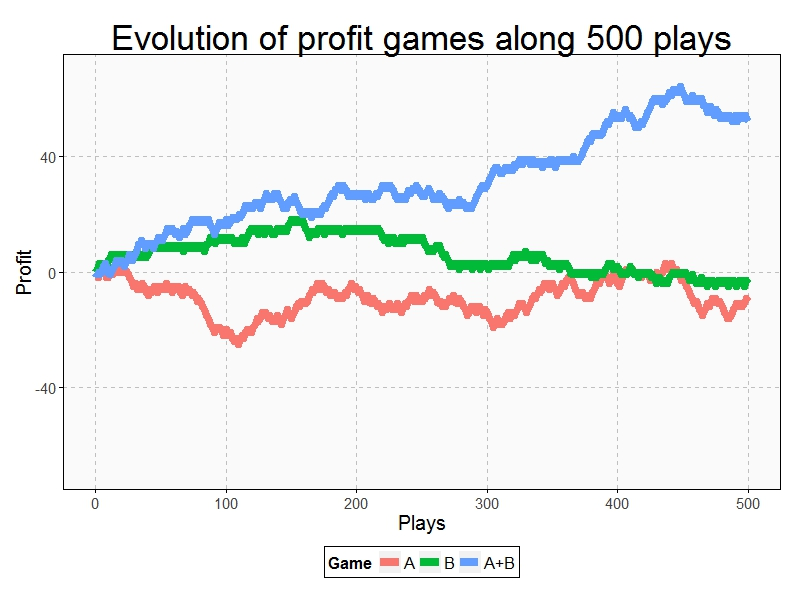Resulting profit of Game A+B after 500 plays  is +52€ and is -9€ and -3€ for Games A and B respectively. Let’s do some more simulations (I removed legends and titles but colors of games are the same):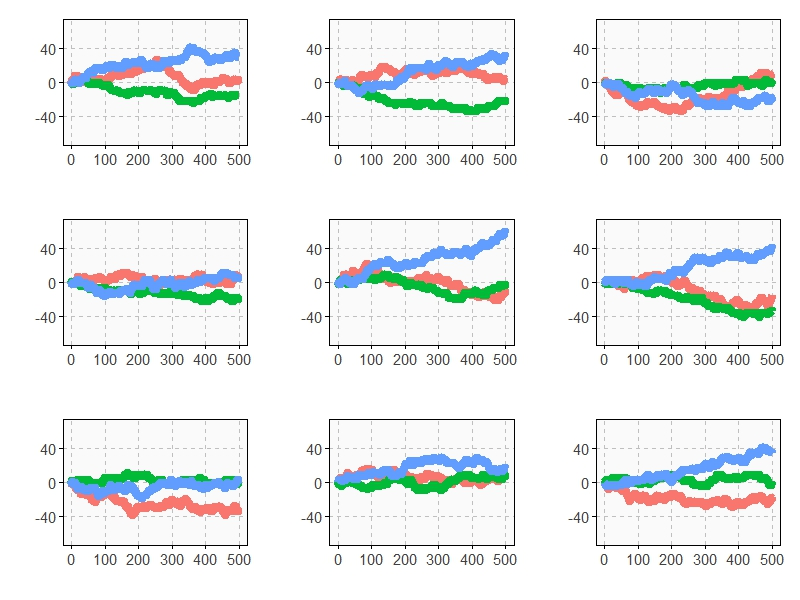As you can see, Game A+B is the most profitable in almost all the previous simulations. Coincidence? Not at all. This is a consequence of the stunning Parrondo’s Paradox which states that two losing games can combine into a winning one.

If you still don’t believe in this brain-crashing paradox, following you can see the empirical distributions of final profits of three games after 1.000 plays: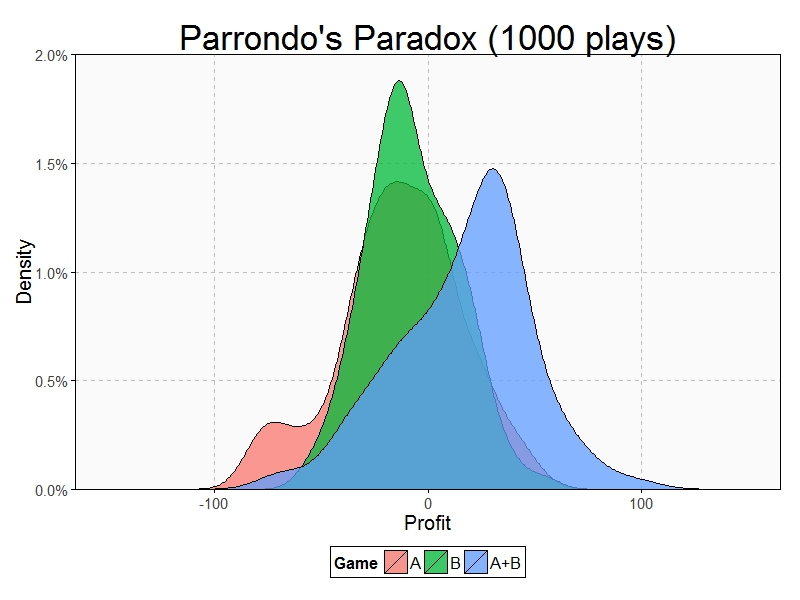After 1000 plays, mean profit of Game A is -13€, is -7€ for Game B and 17€ for Game A+B.

This paradox was discovered in the last nineties by the Spanish physicist Juan Parrondo and can help to explain, among other things, why investing in losing shares can result in obtaining big profits. Amazing:

require(ggplot2)
require(scales)
library(gridExtra)
opts=theme(
legend.position = "bottom",
legend.background = element_rect(colour = "black"),
panel.background = element_rect(fill="gray98"),
panel.border = element_rect(colour="black", fill=NA),
axis.line = element_line(size = 0.5, colour = "black"),
axis.ticks = element_line(colour="black"),
panel.grid.major = element_line(colour="gray75", linetype = 2),
panel.grid.minor = element_blank(),
axis.text.y = element_text(colour="gray25", size=15),
axis.text.x = element_text(colour="gray25", size=15),
text = element_text(size=20),
plot.title = element_text(size = 35))
PlayGameA = function(profit, x, c) {if (runif(1) < c-x) profit+1 else profit-1}
PlayGameB = function(profit, x1, c1, x2, c2) {if (profit%%3>0) PlayGameA(profit, x=x1, c=c1) else PlayGameA(profit, x=x2, c=c2)}
####################################################################
#EVOLUTION
####################################################################
noplays=500
alpha=0.005
profit0=0
results=data.frame(Play=0, ProfitA=profit0, ProfitB=profit0, ProfitAB=profit0)
for (i in 1:noplays) {results=rbind(results, c(i,
PlayGameA(profit=results[results$Play==(i-1),2], x =alpha, c =0.5), PlayGameB(profit=results[results$Play==(i-1),3], x1=alpha, c1=0.75, x2=alpha, c2=0.1),
if (runif(1)<0.5) PlayGameA(profit=results[results$Play==(i-1),4], x =alpha, c =0.5) else PlayGameB(profit=results[results$Play==(i-1),4], x1=alpha, c1=0.75, x2=alpha, c2=0.1)
))}
results=rbind(data.frame(Play=results$Play, Game="A", Profit=results$ProfitA),
data.frame(Play=results$Play, Game="B", Profit=results$ProfitB),
data.frame(Play=results$Play, Game="A+B", Profit=results$ProfitAB))
ggplot(results, aes(Profit, x=Play, y=Profit, color = Game)) +
scale_x_continuous(limits=c(0,noplays), "Plays")+
scale_y_continuous(limits=c(-75,75), expand = c(0, 0), "Profit")+
labs(title="Evolution of profit games along 500 plays")+
geom_line(size=3)+opts
####################################################################
#DISTRIBUTION
####################################################################
noplays=1000
alpha=0.005
profit0=0
results2=data.frame(Play=numeric(0), ProfitA=numeric(0), ProfitB=numeric(0), ProfitAB=numeric(0))
for (j in 1:100) {results=data.frame(Play=0, ProfitA=profit0, ProfitB=profit0, ProfitAB=profit0)
for (i in 1:noplays) {results=rbind(results, c(i,
PlayGameA(profit=results[results$Play==(i-1),2], x =alpha, c =0.5), PlayGameB(profit=results[results$Play==(i-1),3], x1=alpha, c1=0.75, x2=alpha, c2=0.1),
if (runif(1)<0.5) PlayGameA(profit=results[results$Play==(i-1),4], x =alpha, c =0.5) else PlayGameB(profit=results[results$Play==(i-1),4], x1=alpha, c1=0.75, x2=alpha, c2=0.1)))}
results2=rbind(results2, results[results$Play==noplays, ])} results2=rbind(data.frame(Game="A", Profit=results2$ProfitA),
data.frame(Game="B", Profit=results2$ProfitB), data.frame(Game="A+B", Profit=results2$ProfitAB))
ggplot(results2, aes(Profit, fill = Game)) +
scale_x_continuous(limits=c(-150,150), "Profit")+
scale_y_continuous(limits=c(0,0.02), expand = c(0, 0), "Density", labels = percent)+
geom_density(alpha=.75)+opts


# The World We Live In #3: Breastfeeding

Facts are stubborn, but statistics are more pliable (Mark Twain)

According to World Health Organization, exclusive breastfeeding is recommended up to 6 months of age, with continued breastfeeding along with appropriate complementary foods up to two years of age or beyond. Thus, the defining characteristic of continued breastfeeding is that the infant between 6 months and 2 years of age receives at least some breast milk regardless of the quantity or the presence of other foods or liquids in the diet.

On the other hand, as can be read in The World Factbook of Central Intelligence Agency, the Total Fertility Rate (TFR) is the average number of children that would be born to a woman over her lifetime if she were to experience the exact current age-specific fertility rates through her lifetime and she were to survive from birth through the end of her reproductive life. It is obtained by summing the single-year age-specific rates at a given time.

This is how the world is arranged according to these two rates:There are many differences between countries. Both rates are very low in some east European countries like Ukraine, Bosnia, Belarus and Moldova. On the other hand both of them are very high in Benin, Rwanda, Burkina Faso and Malawi, all of them African. Also African countries are Angola, Nigeria and Somalia where fertility rate is very high but breastfeeding is not very established (Timor-Leste in Asia belongs to this segment as well); and women in Nepal, Bangladesh, Sri-Lanka and India feed their moderate number of descendants with their own milk.

We live in a complex and beautiful world which cannot be measured only with averages nor standard deviations:

#Continued breastfeeding rate: http://data.un.org/Data.aspx?d=SOWC&f=inID%3a89
#Total fertility rate (TFR): http://data.un.org/Data.aspx?d=SOWC&f=inID%3a127
#Population: http://data.un.org/Data.aspx?d=SOWC&f=inID%3a105
require("sqldf")
require("ggplot2")
require("scales")
colnames(breastfeeding)="Country"
colnames(fertility)="Country"
colnames(population)="Country"
data=sqldf("SELECT a.Country, a.Value as Pop, b.Value as Fertility, c.Value as Breastfeeding
FROM population a inner join fertility b
on (a.Country=b.Country) INNER JOIN breastfeeding c
on (a.Country=c.Country)
where a.Subgroup = 'Total' AND b.Year = 2011
AND a.Country NOT IN ('World', 'South Asia',
'Sub-Saharan Africa', 'Least Developed Countries/Territories', 'Eastern and Southern Africa',
'East Asia and Pacific')")
opts=theme(
panel.background = element_rect(fill="gray98"),
panel.border = element_rect(colour="black", fill=NA),
axis.line = element_line(size = 0.5, colour = "black"),
axis.ticks = element_line(colour="black"),
panel.grid.major = element_line(colour="gray75", linetype = 2),
panel.grid.minor = element_blank(),
axis.text.y = element_text(colour="gray25", size=15),
axis.text.x = element_text(colour="gray25", size=15),
text = element_text(size=20),
legend.key = element_blank(),
legend.position = "none",
legend.background = element_blank(),
plot.title = element_text(size = 45))
ggplot(data, aes(x=Fertility, y=Breastfeeding/100, size=log(Pop), label=Country), guide=FALSE)+
geom_point(colour="white", fill="darkorchid2", shape=21, alpha=.55)+
scale_size_continuous(range=c(2,40))+
scale_x_continuous(limits=c(1,7))+
scale_y_continuous(limits=c(0,1), labels = percent)+
labs(title="The World We Live In #3: Breastfeeding",
x="Total fertility rate (TFR)",
y="Continued breastfeeding rate")+
geom_text(data=subset(data, Fertility>5 & (Breastfeeding>75|Breastfeeding<40)), size=5.5, colour="gray25", hjust=0, vjust=0)+
geom_text(data=subset(data, Fertility<3 & Breastfeeding>75), size=5.5, colour="gray25", hjust=0, vjust=0)+
geom_text(data=subset(data, Fertility<2 & Breastfeeding<12), size=5.5, colour="gray25", hjust=0, vjust=0)+
geom_text(aes(5, 0), colour="gray25", hjust=0, label="Source: United Nations (size of bubble depending on population)", size=4)+opts


# A Little Present For Coldplay

Gravity, release me, and don’t ever hold me down, now my feet won’t touch the ground (Coldplay, Life In Technicolor II)

Inspired by this nice post and by this cover of a Coldplay’s album: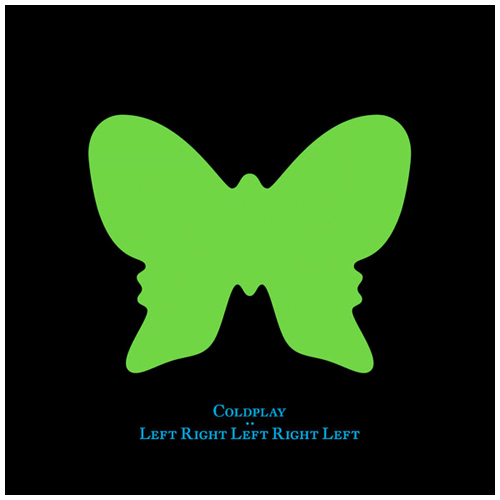I have dared to do this using ggplot, polar coordinates and Google Fonts:Coldplay: feel free to use it for some future album.

library(ggplot2)
library(extrafont)
windowsFonts(Monoton=windowsFont("Monoton"))
butterfly=function(x) 8-sin(x)+2*sin(3*x)+2*sin(5*x)-sin(7*x)+3*cos(2*x)-2*cos(4*x)
opt=theme(legend.position="none",
panel.background = element_rect(fill="black"),
panel.grid = element_blank(),
axis.ticks=element_blank(),
axis.title=element_blank(),
axis.text =element_blank())
ggplot(data.frame(x = c(0, 2*pi)), aes(x)) +
stat_function(fun=butterfly, geom="density", fill="#FC0C54", colour="#FC0C54") +
coord_polar(start=-pi)+
geom_text(x=.5, y=-14, colour="turquoise2", family="Monoton", label="COLDPLAY", size=12)+
geom_text(x=1.5, y=14, colour="turquoise2", family="Monoton", angle=90, label="Up Down Up Down Up Down", size=6)+
opt